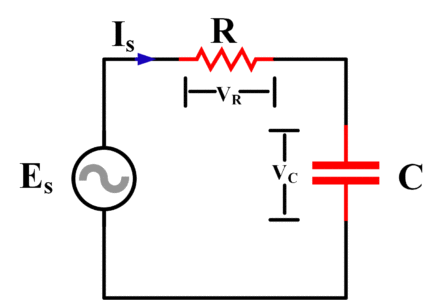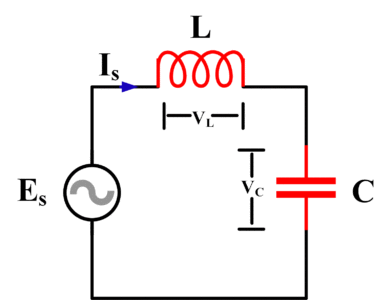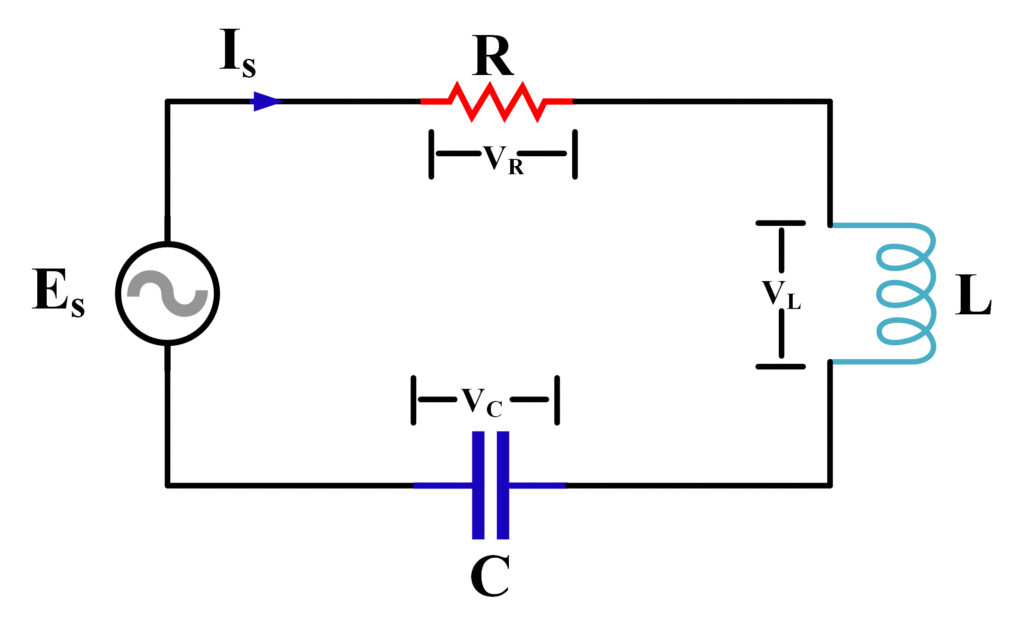Home / Basic Electrical / Passive Components in AC Circuits with Equations

Passive Components in AC Circuits with Equations

Want create site? Find Free WordPress Themes and plugins.

There are three key passive elements used in many electrical and electronic circuits such as: Resistor, Inductor, and Capacitor. All these three elements limit the current flow but in a dissimilar manner.

Since passive elements exhaust energy, they cannot amplify the electrical signals power employed to them and will always possess a gain of a value smaller than one.

Resistors whether employed in AC or DC electric circuits will have the same resistance regardless of the value of the source frequency. This is because resistors possess the parasitic characteristics of like zero inductance and infinite capacitance. In case of resistive circuits, the voltage and current remain in-phase with each other and the power dissipated is the product of the voltage and the current.

Inductors and Capacitors offer a different AC resistance which is commonly known as reactance. The reactance value for an inductor or a capacitor is NOT a fixed value (like a resistance value in case of resistors) but varies as source frequency.

These three passive elements are employed in the electric circuits in several configurations (i.e., series, parallel, or combination of both) to determine different circuit parameters like current, voltage, or component values.

Pure Resistive Circuit

Resistance is a measure of opposition in the current flow in an electric circuit and is measured in Ohm. It can be either of fixed or variable value.Fig.1: Pure Resistive Circuit

Resistive Circuit Equations

${{I}_{s}}=\frac{{{E}_{s}}}{R}$

$Z=R=\frac{{{V}_{R}}}{{{I}_{R}}}$

$Z=R+jX=R+j0=\angle {{0}^{o}}$

Pure Inductive Circuit

Inductance is the property of an element to store energy in a magnetic field and is measured in Henry. At DC, the inductor has zero impedance because of zero frequency whereas at AC (at higher frequencies), it shows an infinite impedance.Fig.2: Pure Inductive Circuit

Inductive Circuit Equations

${{I}_{s}}=\frac{{{E}_{s}}}{{{X}_{L}}}$

${{X}_{L}}=\frac{{{V}_{L}}}{{{I}_{L}}}=2\pi fL$

$Z=R+j{{X}_{L}}=0+j{{X}_{L}}=\angle +{{90}^{o}}$

Pure Capacitive Circuit

The property of a circuit that permits the storage of an electric charge when a difference of voltage is applied. At DC, the capacitor has infinite impedance because of zero frequency whereas at AC (at higher frequencies), it shows zero impedance.Fig.3: Pure Capacitive Circuit

Capacitive Circuit Equations

${{I}_{s}}=\frac{{{E}_{s}}}{{{X}_{C}}}$

${{X}_{C}}=\frac{{{V}_{C}}}{{{I}_{C}}}=\frac{1}{2\pi fC}$

$Z=R-j{{X}_{C}}=0-j{{X}_{C}}=\angle -{{90}^{o}}$

Series AC Circuits

Passive components can be connected in various series combinations to form RL, RC, and LC circuits as described below.

Series RL CircuitFig.4: Series RL Circuit

Series RL Circuit Equations

${{I}_{s}}=\frac{{{E}_{s}}}{Z}=\frac{{{E}_{s}}}{R+j{{X}_{L}}}=\frac{{{E}_{s}}}{\sqrt{{{R}^{2}}+X_{L}^{2}}}$

${{E}_{s}}=\sqrt{V_{R}^{2}+V_{L}^{2}}$

$Z=R+j{{X}_{C}}=\sqrt{{{R}^{2}}+X_{L}^{2}}$

$Z=R+j{{X}_{C}}=\angle +\theta$

Where

${{\theta }_{\left( {{0}^{o}}\to {{90}^{o}} \right)}}={{\tan }^{-1}}\left( \frac{{{X}_{L}}}{R} \right)$

Series RC CircuitFig.5: Series RC Circuit

Series RC Circuit Equations

${{I}_{s}}=\frac{{{E}_{s}}}{Z}=\frac{{{E}_{s}}}{R-j{{X}_{C}}}=\frac{{{E}_{s}}}{\sqrt{{{R}^{2}}+X_{C}^{2}}}$

${{E}_{s}}=\sqrt{V_{R}^{2}+V_{L}^{2}}$

$Z=R-j{{X}_{C}}=\sqrt{{{R}^{2}}+X_{C}^{2}}$

$Z=R-j{{X}_{C}}=\angle -\theta$

Where

${{\theta }_{\left( -{{90}^{o}}\to {{0}^{o}} \right)}}={{\tan }^{-1}}\left( -\frac{{{X}_{C}}}{R} \right)$

Series LC CircuitFig.6: Series LC Circuit

Series LC Circuit Equations

${{I}_{s}}={{I}_{L}}={{I}_{C}}$

Resonance frequency

${{f}_{R}}=\frac{1}{2\pi \sqrt{LC}}$

At resonance frequency, we observe the following expressions

\begin{align} & 1.{{X}_{L}}={{X}_{C}} \\ & 2.{{V}_{L}}={{V}_{C}} \\\end{align}

${{E}_{s}}=\sqrt{V_{R}^{2}+V_{L}^{2}}$

$Z=R+jX=0+j\left( {{X}_{L}}-{{X}_{C}} \right)$

Or

$Z=R+jX=0+j\left( {{X}_{C}}-{{X}_{L}} \right)$

In another form,

$Z=\sqrt{X_{L}^{2}-X_{C}^{2}}$

Parallel AC Circuits

Passive components can be connected in various parallel combinations to form RL, RC, and LC circuits as described below.

Parallel RL CircuitFig.7: Parallel RL Circuit

Parallel RL Circuit Equations

${{I}_{R}}=\frac{{{E}_{s}}}{R}$

${{I}_{L}}=\frac{{{E}_{s}}}{{{X}_{L}}}$

${{I}_{s}}=\sqrt{I_{R}^{2}+I_{L}^{2}}$

$\theta ={{\tan }^{-1}}\left( \frac{{{I}_{L}}}{{{I}_{R}}} \right)$

${{E}_{s}}={{V}_{R}}={{V}_{L}}$

Parallel RC CircuitFig.8: parallel RC Circuit

Parallel RC Circuit Equations

${{I}_{R}}=\frac{{{E}_{s}}}{R}$

${{I}_{C}}=\frac{{{E}_{s}}}{{{X}_{C}}}$

${{I}_{s}}=\sqrt{I_{R}^{2}+I_{C}^{2}}$

$\theta ={{\tan }^{-1}}\left( \frac{{{I}_{C}}}{{{I}_{R}}} \right)$

${{E}_{s}}={{V}_{R}}={{V}_{C}}$

Parallel LC CircuitFig.9: Parallel LC Circuit

Parallel LC Circuit Equations

$Y=\frac{1}{Z}={{B}_{L}}+{{B}_{C}}$

Where

\begin{align} & {{B}_{L}}=\frac{1}{{{X}_{L}}} \\ & {{B}_{C}}=\frac{1}{{{X}_{C}}} \\\end{align}

Resonance frequency

${{f}_{R}}=\frac{1}{2\pi \sqrt{LC}}$

${{E}_{s}}={{V}_{L}}={{V}_{C}}$

At resonance frequency, we observe the following expressions

\begin{align} & 1.{{X}_{L}}={{X}_{C}} \\ & 2.{{I}_{L}}={{I}_{C}} \\\end{align}

Passive RLC AC Circuits

All three passive components can be connected together to form series PLC and parallel RLC combinations as described below.

Series RLC CircuitFig.10: Series RLC Circuit

Series RLC Circuit Equations

${{I}_{s}}={{I}_{R}}={{I}_{L}}={{I}_{C}}$

${{I}_{s}}=\frac{{{E}_{s}}}{Z}=\frac{{{E}_{s}}}{\sqrt{{{R}^{2}}+{{\left( {{X}_{L}}-{{X}_{C}} \right)}^{2}}}}$

$Z=R+jX=\angle \theta$

$Z=\sqrt{{{R}^{2}}+{{\left( {{X}_{L}}-{{X}_{C}} \right)}^{2}}}$

${{E}_{s}}=\sqrt{V_{R}^{2}+{{\left( {{V}_{L}}-{{V}_{C}} \right)}^{2}}}$

$\theta ={{\tan }^{-1}}\left( \frac{{{X}_{L}}-{{X}_{C}}}{R} \right)$

Parallel RLC CircuitFig.11: Parallel RLC Circuit

Parallel RLC Circuit Equations

$Y=\frac{1}{Z}=\sqrt{{{G}^{2}}+{{\left( {{B}_{L}}-{{B}_{C}} \right)}^{2}}}$

Where

$G=\frac{1}{R}$

${{B}_{L}}=\frac{1}{{{X}_{L}}}$

${{B}_{C}}=\frac{1}{{{X}_{C}}}$

${{I}_{s}}=\sqrt{I_{R}^{2}+{{\left( {{I}_{L}}-{{I}_{C}} \right)}^{2}}}$

Resonance frequency

${{f}_{R}}=\frac{1}{2\pi \sqrt{LC}}$

${{E}_{s}}={{V}_{R}}={{V}_{L}}={{V}_{C}}$

From above analysis, it is evident that behavior of passive components is dissimilar in AC and DC Circuits because of the frequency involvement. In a strictly resistive circuit, the voltage and current are in phase with each other. In a strictly inductive circuit, current lags the voltage by 90o while in a strictly capacitive circuit, current leads the voltage by 90o.

In a series circuit, the current flow through each component remains the same and the source voltage equals the phasor sum of the voltages across the different passive components. In a parallel circuit, the voltage across each component remains the same and the source current equals the phasor sum of the currents in each circuit branch.

In Series and Parallel RLC circuits, when the source voltage is “in-phase” with the source current, the resonance occurs as XL = XC. A series resonance circuit is commonly called an Acceptor circuit whereas a parallel resonance circuit is commonly called a Rejecter circuit.

Did you find apk for android? You can find new Free Android Games and apps.